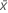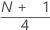# Methods and formulas for Display Descriptive Statistics

Select the method or formula of your choice.

## Mean

A commonly used measure of the center of a batch of numbers. The mean is also called the average. It is the sum of all observations divided by the number of (nonmissing) observations.

### Notation

TermDescription
xiith observation
Nnumber of nonmissing observations

## Standard error of the mean (SE Mean)

The standard error of the mean is calculated as the standard deviation divided by the square root of the sample size.

### Notation

TermDescription
s standard deviation of the sample
N number of nonmissing observations

## Standard deviation (StDev)

The sample standard deviation provides a measure of the spread of your data. It is equal to the square root of the sample variance.

### Formula

If the column contains x 1, x 2,..., x N, with mean, then the standard deviation of the sample is:

### Notation

TermDescription
x i i th observationmean of the observations
N number of nonmissing observations

## Variance

The variance measures how spread out the data are about their mean. The variance is equal to the standard deviation squared.

### Notation

TermDescription
xiith observationmean of the observations
Nnumber of nonmissing observations

## Coefficient of variation (CoefVar)

The coefficient of variation is a measure of relative variability calculated as a percentage.

### Formula

Minitab calculates it as:

### Notation

TermDescription
s standard deviation of the samplemean of the observations

## 1st quartile (Q1)

25% of your sample observations are less than or equal to the value of the 1st quartile. Therefore, the 1st quartile is also referred to as the 25th percentile.

### Notation

TermDescription
ytruncated integer value of w
wzfraction component of w that was truncated
xjjth observation in the list of sample data, ordered from smallest to largest
###### Note

When w is an integer, y = w, z = 0, and Q1 = xy.

## Median

The sample median is in the middle of the data: at least half the observations are less than or equal to it, and at least half are greater than or equal to it.

Suppose you have a column that contains N values. To calculate the median, first order your data values from smallest to largest. If N is odd, the sample median is the value in the middle. If N is even, the sample median is the average of the two middle values.

For example, when N = 5 and you have data x1, x2, x3, x4, and x5, the median = x3.

When N = 6 and you have ordered data x1, x2, x3, x4, x5,and x6:

where x3 and x4 are the third and fourth observations.

## 3rd quartile (Q3)

75% of your sample observations are less than or equal to the value of the third quartile. Therefore, the third quartile is also referred to as the 75th percentile.

### Notation

TermDescription
ytruncated value of w
w
zfraction component of w that was truncated away
xjjth observation in the list of sample data, ordered from smallest to largest
###### Note

When w is an integer, y = w, z = 0, and Q3 = xy.

## Interquartile range (IQR)

The interquartile range equals the third quartile minus the 1st quartile.

## Mode

The mode is the data value that occurs most often in the dataset. If multiple modes exist, Minitab displays the smallest modes, up to a total of four. N for Mode is the number of times the mode (or modes) appears.

## Trimmed mean (TrMean)

Minitab calculates the trimmed mean by removing the smallest 5% and the largest 5% of the values (rounded to the nearest integer), and then calculating the mean of the remaining values.

## Sum

### Notation

TermDescription
xi i th observation

## Minimum

The smallest value in your data set.

## Maximum

The largest value in your data set.

## Range

The range is calculated as the difference between the largest and smallest data value.

R = Maximum – Minimum

## Sum of squares

Minitab squares each value in the column, then computes the sum of those squared values.

### Notation

TermDescription
xi i th observation

## Skewness

Skewness is a measure of asymmetry. A negative value indicates skewness to the left, and a positive value indicates skewness to the right. A zero value does not necessarily indicate symmetry.

### Notation

TermDescription
xi i th observationmean of the observations
N number of nonmissing observations
s standard deviation of the sample

## Kurtosis

Kurtosis is one measure of how different a distribution is from the normal distribution. A positive value usually indicates that the distribution has a sharper peak than the normal distribution. A negative value indicates that the distribution has a flatter peak than the normal distribution.

### Notation

TermDescription
xi i th observationmean of the observations
N number of nonmissing observations
s standard deviation of the sample

## MSSD (mean of the squared successive differences)

Minitab calculates half the MSSD (mean of the squared successive differences) of a batch of numbers. The successive differences are squared and summed. Then Minitab divides by 2 and calculates the average.

### Notation

TermDescription
xi i th observationmean of the observations

## N nonmissing (N)

The number of non-missing values in the sample.

## N missing (N*)

The number of missing values in the sample. The number of missing values refers to cells that contain the missing value symbol *.

## N total (total count)

The total number of observations in the column.

## Percent

Minitab calculates what percentage of the whole that is accounted for by each group.

### Notation

TermDescription
ninumber of observations in the ith group
Nnumber of nonmissing observations

## Cumulative percent (CumPct)

Minitab calculates the cumulative percentage that is represented by each group.

### Notation

TermDescription
ninumber of observations in the ith group
Nnumber of nonmissing observations
By using this site you agree to the use of cookies for analytics and personalized content.  Read our policy